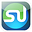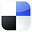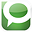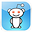# ANOVA TestDone in ExcelCompared To By Hand

ANOVA is something you would do by hand, ONLY if you absolutely had to. I remember being forced to perform these calculations by hand in statistics class when I was getting my MBA. I also remember wondering why in the world we were doing that when we all knew Excel. Still don’t have an answer to that question. It seemed a little like doing multiplication on a slide rule even though a calculator was readily available. My father once showed me his old slide rule and how facile he had become with it. It was almost magic. I never got the urge to figure it out though. Sorry Dad.

The video below shows a statistical procedure called Single-Factor ANOVA (the simplest type of ANOVA) being solved in Excel and then solved by hand. I’m hoping that the torturous hand calculations in the video only serve to more strongly contrast the ease of doing statistics in Excel.

Step-By-Step Video Showing How To Do Single-Factor ANOVA In Excel and Also How To Do It By Hand:

Why Excel Is A Good Starting Point To Teach Statistics

The point of this article and the linked video is to persuade statistics teachers to focus their efforts on using tools like Excel right from the start. Slogging through those hand calculations is almost never a good thing. Probably the fastest way to make a statistics student to seriously hate statistics is to force him or her to do calculation-intensive tasks like regression and ANOVA by hand.

As an Internet marketing manager who does a lot of statistics on the job, I am SOOO glad I learned how to do all of my statistic procedures on Excel. Believe it or not, statistics is actually kind of fun when you have such a convenient tool like Excel (I’ve been called a Propeller Head more than once). There are plenty of other fine statistical tools like Minitab, SPSS, and SAS. But….I (like almost any other business manager) have a pretty good grasp of Excel. Honestly, I don’t really have no desire to learn SAS (or any other statistical software that costs thousands of dollars) when I’ve got Excel. If I can use Excel instead, you bet I’m gonna.

Here’s a little info about the ANOVA test that was run in the above video:

What Is ANOVA?

ANOVA stand for Analysis of Variance. It is a test to determine if three or more variations of each of one or more factors have an effect on a population. ANOVA tests the Null Hypothesis of each factor. The Null Hypothesis of each factor states that varying the factor has no effect on a population. The ANOVA test results in either acceptance or rejection of the Null Hypothesis within a specified degree of certainty.

The single-factor ANOVA test in the linked video evaluates whether different closing methods affect the probability that a sale will close. The Null Hypothesis states that varying the closing method does not affect the number of sales that get closed. All other factors, including the abilities of the individual salespeople, are assumed to be the same.

The Null Hypothesis

Acceptance or rejection of the Null Hypothesis can be determined by either the P Value or the F Statistic obtained by the calculations. Both the P Value and the F Statistic are equivalent to each other and are nearly interchangeable. The video provide a detailed explanation of the following: We accept the Null Hypothesis if the P Value is greater than Alpha (Alpha = 1 – Required Degree of Certainty) or, equivalently, if the calculated F Statistics is less than F Critical. We reject the Null Hypothesis if the opposite is true. Rejection of the Null Hypothesis implies that variation of the associated factor did affect the outcome.

Doing ANOVA By Hand vs. By Excel

Doing ANOVA in Excel takes just a few seconds with little possibility of error if the data is inserted correctly. Doing ANOVA by hand takes a LONG time and has LOTS of opportunities for error. Here, the above video of step-by-step ANOVA video will, hopefully, will convince you of that.

Here Is the Original Problem to Be Solved With Single-Factor ANOVA:
Click On Image To See Enlarged View

A group of 4 salespeople used a different closing method exclusively each week for 3 weeks. The sales totals for each salesperson using each method are shown above. We need to determine within 95% certainty whether varying the closing method affected sales numbers or not. No other factors were varied during the 3-week duration of this test.

Here is the Problem Solved in Excel in One Step:

Click On Image To See Enlarged View

The Excel output shows the P Value associated with the closing methods to be 0.0144. This is significantly less than the alpha of 0.05, so we can reject the Null Hypothesis and state with 95% that varying the closing method did affect sales totals. Remember, it took less than 10 seconds to input the data from the excel spread sheet and get the above output. Compare this to doing the same problem by hand below and arriving at the same answer.

Now, Here is The Same Problem Done By Hand

Click On Image To See Enlarged View

Click On Image To See Enlarged View

Click On Image To See Enlarged View

Click On Image To See Enlarged View

Hopefully this article touched a nerve with the poor folks out there who were forced to do ANOVA by hand in statistics class. There might even be a few statistics teachers who would rather have taught ANOVA in Excel than having had to do it by hand in front of the class room. I've had to teach ANOVA by hand to a class or two and it wasn't the funnest thing I've ever done.

If You Like This, Then Share It...Excel Master Series Blog Directory

Statistical Topics and Articles In Each Topic

• Histograms in Excel
• Bar Chart in Excel
• Combinations & Permutations in Excel
• Normal Distribution in Excel
• t-Distribution in Excel
• Binomial Distribution in Excel
• z-Tests in Excel
• t-Tests in Excel
• Hypothesis Tests of Proportion in Excel
• Chi-Square Independence Tests in Excel
• Chi-Square Goodness-Of-Fit Tests in Excel
• F Tests in Excel
• Correlation in Excel
• Pearson Correlation in Excel
• Spearman Correlation in Excel
• Confidence Intervals in Excel
• Simple Linear Regression in Excel
• Multiple Linear Regression in Excel
• Logistic Regression in Excel
• Single-Factor ANOVA in Excel
• Two-Factor ANOVA With Replication in Excel
• Two-Factor ANOVA Without Replication in Excel
• Creating Interactive Graphs of Statistical Distributions in Excel
• Solving Problems With Other Distributions in Excel
• Optimization With Excel Solver
• Chi-Square Population Variance Test in Excel
• Analyzing Data With Pivot Tables
• SEO Functions in Excel
• Time Series Analysis in Excel

1.AWESOME AWESOME AWESOME ARTICLE THANK YOU SO MUCH!!! Very helpful.

2.I GET IT! Thank you.

3.One more thing that I desire to share at this place is that, doesn’t matter what you are using free blogging service except if you don’t update your blog on regularly basis then it’s no more worth.

---------------------
Best website design and development Company In Kanpur